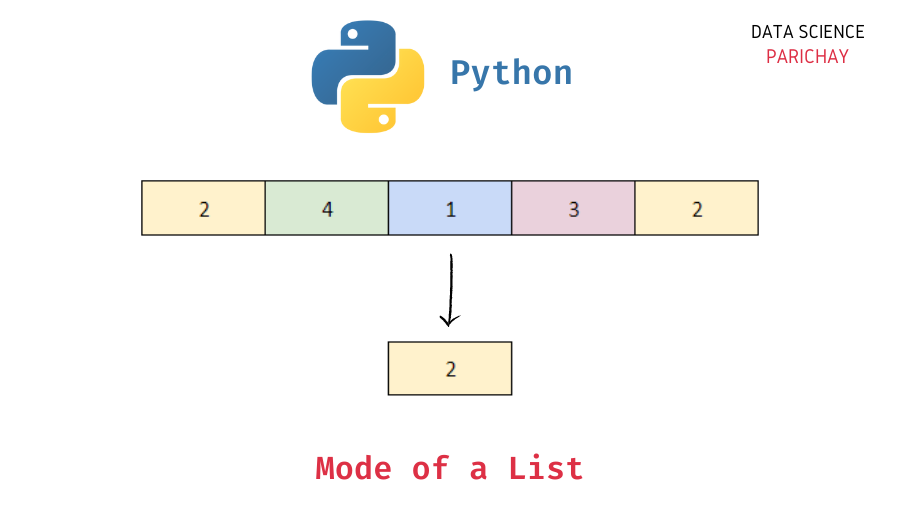# Find Mode of List in Python

In this tutorial, we will look at how to calculate the mode of a list in Python with the help of some exmaples.

Mode is a descriptive statistic that is used as a measure of central tendency of a distribution. It is equal to value that occurs the most frequently. Note that it’s possible for a set of values to have more than one mode. Mode is also used to impute missing value in categorical variables.

To calculate the mode of a list of values –

1. Count the frequency of each value in the list.
2. The value with the highest frequency is the mode.

For example, calculate the mode of the following values –

If we count how many times each value occurs in the list, you can see that 2 occurs three times, 5 occurs two times and 3, 4, and 6 occur one time each. From this we can say that the mode of these numbers is 2.

Let’s look at another example.

2 and 5 occur two times and 1, 3, 4, and 6 occur once. Here, both 2 and 5 are the modes as they both have the highest frequency of occurrence. A distribution with two modes is called a bimodal distribution.

To compute the mode of a list of values in Python, you can write your own custom function or use methods available in other libraries such as `scipy`, `statistics`, etc. Let’s look at these methods with the help of some examples.

📚 Data Science Programs By Skill Level

Introductory

Intermediate ⭐⭐⭐

🔎 Find Data Science Programs 👨‍💻 111,889 already enrolled

Disclaimer: Data Science Parichay is reader supported. When you purchase a course through a link on this site, we may earn a small commission at no additional cost to you. Earned commissions help support this website and its team of writers.

We already know the logic to compute the mode. Let’s now implement that logic in a custom Python function.

```def mode(ls):
# dictionary to keep count of each value
counts = {}
# iterate through the list
for item in ls:
if item in counts:
counts[item] += 1
else:
counts[item] = 1
# get the keys with the max counts
return [key for key in counts.keys() if counts[key] == max(counts.values())]

# use the function on a list of values
mode([2,2,4,5,6,2,3,5])```

Output:

``

Here, you can see that the custom function gives the correct mode for the list of values passed. Note that the function returns a list of all the modes instead of a scaler value.

Let’s now pass a list of values that has two modes.

```# two values with max frequency
mode([2,2,4,5,6,1,3,5])```

Output:

`[2, 5]`

You can see that it returns both the modes as a list. We can modify the function to return a scaler value, for example, the smallest mode or the largest mode depending upon the requirement.

Note that the above implementation may not be the most optimized version. (For instance, you can use `Counter` from the `collections` module to count frequency of values in a list, etc.)

You can also use the `statistics` standard library in Python to get the mode of a list of values. Pass the list as an argument to the `statistics.mode()` function.

```import statistics
# calculate the mode
statistics.mode([2,2,4,5,6,2,3,5])```

Output:

`2`

We get the scaler value 2 as the mode which is correct.

This method gives a `StatisticsError` if there are more than one mode present in the data. For example –

```# calculate the mode
statistics.mode([2,2,4,5,6,1,3,5])```

Output:

```---------------------------------------------------------------------------
StatisticsError                           Traceback (most recent call last)
<ipython-input-20-83fc446343a0> in <module>
1 # calculate the mode
----> 2 statistics.mode([2,2,4,5,6,1,3,5])

~\anaconda3\envs\dsp\lib\statistics.py in mode(data)
505     elif table:
506         raise StatisticsError(
--> 507                 'no unique mode; found %d equally common values' % len(table)
508                 )
509     else:

StatisticsError: no unique mode; found 2 equally common values```

You can also use the `mode()` function available in the `scipy.stats` module to calculate the mode in a list. For example –

```from scipy.stats import mode
# calculate the mode
mode([2,2,4,5,6,2,3,5])```

Output:

`ModeResult(mode=array(), count=array())`

We get the correct result.

Note that this method gives the smallest mode if there are multiple modes present in the data.

```from scipy.stats import mode
# calculate the mode
mode([2,2,4,5,6,1,3,5])```

Output:

`ModeResult(mode=array(), count=array())`

The data actually has two modes, 2 and 5, with both occurring two times but we get 2 as the result because it’s the smallest of the modes.

You can use methods similar to the ones described in this tutorial to calculate the median of a list in Python.

•